# Samacheer Kalvi 6th Maths Solutions Term 3 Chapter 5 Information Processing Additional Questions## Tamilnadu Samacheer Kalvi 6th Maths Solutions Term 3 Chapter 5 Information Processing Additional Questions

Question 1.
Find the figure which is different from others.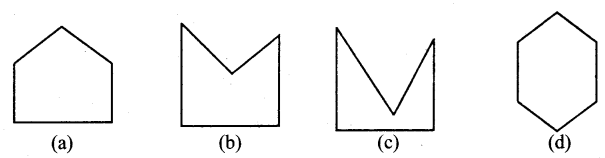Hint:
D is different from other figures.
All other figures has 5 lines and D has 6 lines in it.
Solution:
(d)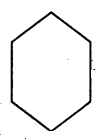Question 2.
If GARIMA is coded as 725432 and TINA as 6482, how will MARTINA be coded?
(a) 3256482
(b) 3265842
(c) 3645862
(d) 3658426
Hint: GARIMA and TINA both have the lettersT and A and they are coded as 4 and 2 repectively.
∴ In MARTINA, I coded as 4 and A coded as 2 in (A) 3256482
Solution:
(a) 3256482

Question 3.
If ‘+’ is ‘×’, is ‘+% ‘×’ is ‘÷’ and ‘÷’ is then answer the following 21 ÷ 8 8 + 2 – 12 × 3 = ?
(a) 14
(b) 9
(c) 13
(d) 11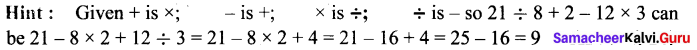Solution:
(a) 14

Question 4.
Which number will replace the question mark?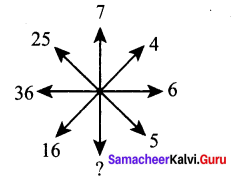(a) 7
(b) 14
(c) 48
(d) 49
Hint: [∴ Left side numbers are square numbers] .
Solution:
(d) 49.Question 5.
Find the correct figure which replaces the ‘?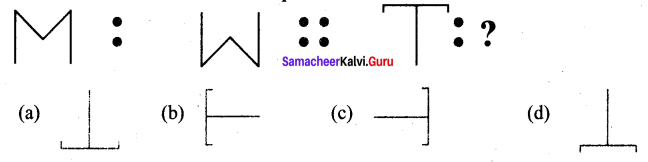Hint: Upside down
Solution: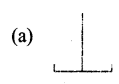Question 6.
Which comes next ?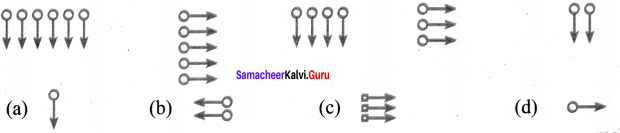Hint : Here the figures rotate clockwise and anticlockwise alternatively making an angle 90° and one arrow deleted in every successive block.
Solution: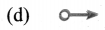Question 7.
Find the number which replaces the question mark in the following series.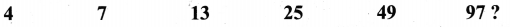(a) 25
(b) 49
(c) 97
(d) 193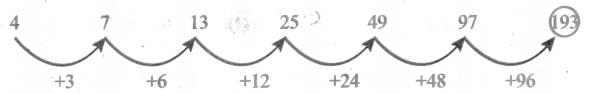Solution:
(d) 193

Question 8.
Identify the number which replaces the question mark?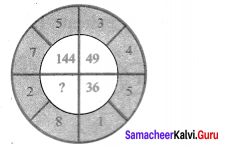(a) 82
(b) 124
(c) 100
(d) 64
Hint: (2 + 8)2 = 102 = 100
Solution:
(c) 100Question 9.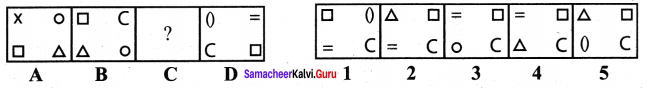Hint: In each step each one of the existing elements moves to the clockwise adjacent
comer. Also in one step, the element that reaches the upper-right comer gets replaced by a new element and in the next step, the element that reaches the lower left comer gets replaced by a new element.
Solution:
(d) 5

Question 10.
1, 6, 15, ?, 45, 66, 91. Find the missing term.
(a) 25
(b) 28
(c) 33
(d) 38
Hint: 1 + 5 = 6, 6 + 9 = 15, so missing term = 15 + 13 = 28
Solution:
(b) 28